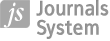RESEARCH PAPER
Human Capital and Economic Growth in the OECD Countries

More details
Hide details
Publication date: 2004-03-25

GNPJE 2004;190(3):16–26

ABSTRACT
In this study, the Mankiw-Romer-Weil model has been modified, so that the steady state of growth was determined by both the physical and human capital expenditures per effective labour unit. To this end, joint expenditures on physical and human capital have been used for estimations. Private and public spending on education at all levels has been taken into account in analyses of expenditures on human capital. Empirical analyses involve the impact of human capital formation on economic growth process in 29 OECD countries in the years 1980-1998. In all the estimated equations the variation in ratios of investment in physical capital had a statistically relevant impact on the variation in GDP per capita growth rates. The estimates of GDP per capita growth rates equations presented in the study suggest a relatively strong conditional convergence effect. In certain equations the ratios of investment in human capital are statistically relevant, while in other equations they are not. In the authors’ opinion this can be explained in two ways. First, the ratios of investment in human capital, measured by private and public expenditures on education are much lower than the ratios of investment in physical capital. Consequently, their impact on economic growth rates may be less pronounced. Second, the ratios of investment in human capital are (as a rule) higher in countries with higher GDP per capita. If the conditional convergence effect is present in OECD countries, this may weaken the impact of ratios of investment in human capital on growth rates of GDP per capita.
 eISSN: 2300-5238